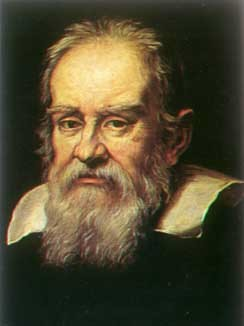https://www.mathed.page/infinity/galileo.html

Visit Henri Picciotto's Math Education Page. Send me e-mail .
Infinity

# Galileo on InfinityGalileo (1564-1642) is best known as the father of experimental physics and modern astronomy. In the excerpt below (from Dialogue on Two New Sciences, 1638) he asks with prophetic clarity the questions at the core of the mathematical and philosophical questions surrounding the concept of infinity. His use of the one-to-one mapping as the key approach to answering these questions foreshadows Cantor's breakthroughs more than two centuries later.

[text below as quoted in Sherman Stein's
Mathematics: The Man-made Universe, 2nd edition, Freeman, 1962;
pp. 314-315.]

Simplicio: Here a difficulty presents itself which appears to me insoluble. Since it is clear that we may have one line segment longer than another, each containing an infinite number of points, we are forced to admit that, within one and the same class, we may have something greater than infinity, because the infinity of points in the long line segment is greater than the infinity of points in the short line segment. This assigning to an infinite quantity a value greater than infinity is quite beyond my comprehension.

Salviati: This is one of the difficulties which arise when we attempt, with our finite minds, to discuss the infinite, assigning to it those properties which we give to the finite and limited; but this I think is wrong, for we cannot speak of infinite quantities as being the one greater or less than or equal to another. To prove this I have in mind an argument, which, for the sake of clearness, I shall put in the form of questions to Simplicio who raised this difficulty.

I take it for granted that you know which of the numbers are squares and which are not.

Simplicio: I am quite aware that a squared number is one which results from the multiplication of another number by itself: thus 4, 9, etc., are squared numbers which come from multiplying 2, 3, etc. by themselves.

Salviati: Very well; and you also know that just as the products are called squares the factors are called roots; while on the other hand those numbers which do not consist of two equal factors are not squares. Therefore if I assert that all numbers, including both squares and non-squares, are more than the squares alone, I shall speak the truth, shall I not?

Simplicio: Most certainly.

Salviati: If I should ask further how many squares there are, one might reply truly that there are as many as the corresponding number of roots, since every square has its own root and every root its own square, while no square has more than one root and no root more than one square.

Simplicio: Precisely so.

Salviati: But if I inquire how many roots there are, it cannot be denied that there are as many as there are numbers because every number is a root of some square. This being granted we must say that there are as many squares as there are numbers because they are just as numerous as their roots, and all the numbers are roots. Yet at the outset we said there are many more numbers than squares, since the larger portion of them are not squares. Not only so, but the proportionate number of squares diminishes as we pass to larger numbers. Thus up to 100 we have 10 squares, that is, the squares constitute 1/10 part of all the numbers; up to 10,000 wefind only 1/100th part to be squares; and up to a million only l/1000th part; on the other hand in an infinite number, if one could conceive of such a thing, he would be forced to admit that there are as many squares as there are numbers all taken together.

Sagredo: What then must one conclude under these circumstances?

Salviati: So far as I see we can only infer that the totality of all numbers is infinite, that the number of squares is infinite, and that the number of their roots is infinite; neither is the number of squares less than the totality of all numbers, nor the latter greater than the former; and finally the attributes "equal," "greater," and "less" are not applicable to infinite, but only to finite, quantities. When, therefore, Simplicio introduces several lines of different lengths and asks me how it is possible that the longer ones do not contain more points than the shorter, I answer him that one line does not contain more or less or just as many points as another, but that each line contains an infinite number. Or if I had replied to him that the points in one line segment were equal in number to the squares; in another, greater than the totality of numbers; and in the little one, as many as the number of cubes, might I not, indeed, have satisfied him by thus placing more points in one line than in another and yet maintaining an infinite number in each? So much for the first difficulty.

Sagredo: Pray stop a moment and let me add to what has already been said an idea which just occurs to me. If the preceding be true, it seems to me impossible to say that one infinite number is greater than another. ...

Infinity
 Visit Henri Picciotto's Math Education Page. Send me e-mail .